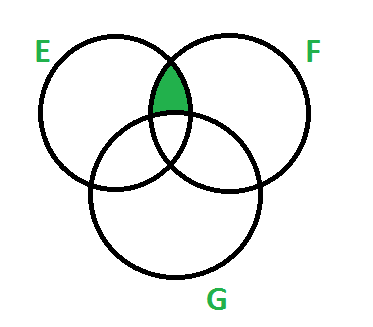Related Articles

# GATE | GATE-CS-2006 | Question 22

• Last Updated : 14 Feb, 2018

Let E, F and G be finite sets.
Let X = (E ∩ F) – (F ∩ G) and Y = (E – (E ∩ G)) – (E – F).
Which one of the following is true?

(A) X ⊂ Y
(B) X ⊃ Y
(C) X = Y
(D) X – Y ≠ φ and Y – X ≠ φ